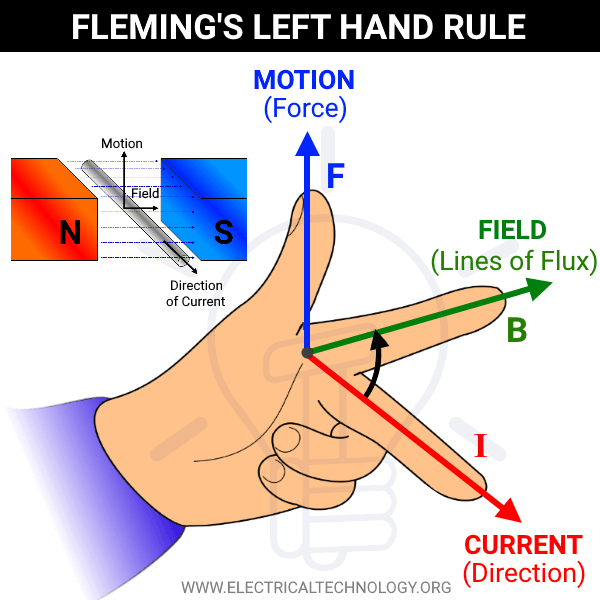# Fleming’s Left Hand Rule and Fleming’s Right Hand Rule

## Fleming’s Left and Right Hand Rules – What is the Difference?

John Ambrose Fleming introduced these useful rules in the late 19th century which are applicable in magnetism and electromagnetism. Both rules can be used to find the direction of the third quantity if the other two are known before.

We know that when a current carrying conductor lays in a magnetic field, a mechanical force applies on the conductor. The direction of this applied force can be found through Fleming’s left hand rule.

Similarly, when a conductor moves in a magnetic field, EMF and current induce in it. The direction of this induced current can be found using Fleming’s right hand rule.

Fleming’s hand rules only show the direction of three related parameters (current, force and magnetic field) i.e. it is not used to find the magnitude of these quantities.

Related Posts:

### Fleming’s Left Hand Rule

Whenever a current carrying conductor lays in a magnetic field, a mechanical force is applied on the conductor to push it away from the field. The value of this mechanical force can be calculated through:

F = BIl     …     Newtons

or

F = μo μr HIl     …     Newtons

Where:

• F = Force in Newtons
• B = Magnetic Flux (flux density)
• I = Current
• l = Length of the conductor
• μo = Absolute permeability
• μr = Relative permeabilityThe direction of this force is opposite to the direction of the current and perpendicular to the direction of the magnetic field.

Fleming’s left hand rule can be used to find the direction of current in the conductor laying in a magnetic field.Fleming’s left hand states that When you keep the thumb, index finger and middle finger of the left hand at right angles (90°) to each other. If the thumb shows the applied force or motion, the first (index or forefinger) shows the lines of flux (field), then the second (middle) finger shows the direction of current.• Good to Know: Fleming’s left hand rule is applicable to electrical motors, That’s why it is also known as Motor Rule.

### Fleming’s Right Hand Rule

According to the Faraday’s law of electromagnetic induction, when a conductor moves in a magnetic field, an EMF is induced in it. The direction of this EMF and current can be defined by Fleming’s right hand rule.Fleming’s right hand rule states that if the thumb, the forefinger and the middle finger are held in such a way that they are mutually perpendicular to each other (makes 90° of angle), then the forefinger points the direction of the field, the thumb points the direction of motion of the conductor and the middle finger points the direction of the induced current (from EMF).• Good to Know: Fleming’s right hand rule is applicable to electrical generators and alternators. That’s why it is also known as generator rule.

Related Posts:

### Differences between Fleming’s Left Hand and Right Hand Rules

 Left Hand Rule Right Hand Rule Used for eclectic motors. Used for electric generators. Used to find the direction of motion and current in electric motors. Used to find the direction of induced EMF & Current in eclectic generators. The thumb shows the direction of applied force or motion. The thumb shows the direction of motion of the conductor in a magnetic field. The first (index of forefinger) shows the direction of lines of magnetic force (flux). The first (index of forefinger) shows the direction of lines of magnetic force (flux). The second (middle) finger shows the direction of current. The second (middle) finger shows the direction of induced EMF and current. The Left hand is used for motors in this rule. The Right hand is used for generators in this rule.Related Posts